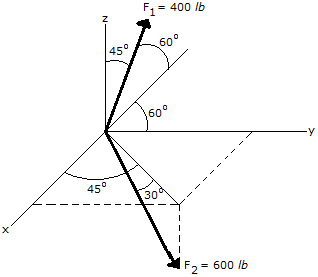# Engineering Mechanics - Force Vectors - Discussion

### Discussion :: Force Vectors - General Questions (Q.No.7)

7.Express the Force F2 in Cartesian vector form.

 [A]. F2 = (155 i + 155 j + 300 k) lb [B]. F2 = (212 i + 212 j - 519 k) lb [C]. F2 = (155 i + 155 j - 300 k) lb [D]. F2 = (367 i + 367 j - 300 k) lb

Explanation:

No answer description available for this question.

 Ahmed said: (Nov 10, 2011) Sir I want explanation for this answer.

 Sarah said: (Aug 21, 2012) Component along z axis: -600 sin 30=-300lb component along x axis: (600 cos 30)(cos 45)= 367.42lb component along y axis: (600 cos 30)(sin 45)= 367.42lb answer: {367 i + 367 j - 300k } lb

 Vinay said: (Jan 26, 2016) Can anyone explain how is component 600 sin 30 will come?